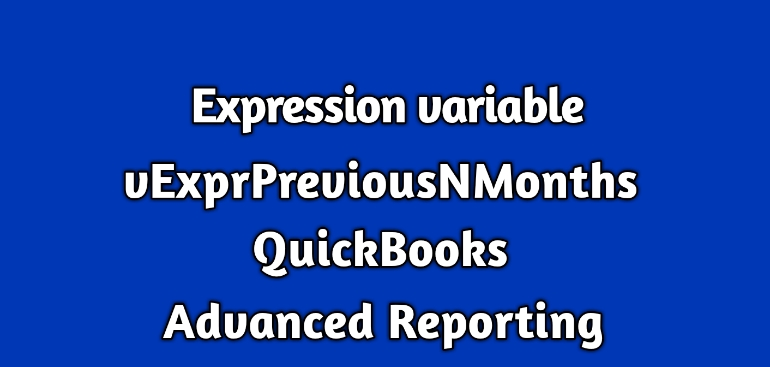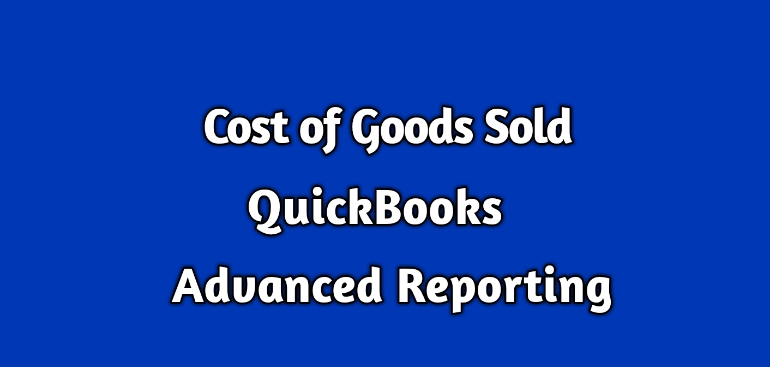## Expression variable (vExprPreviousNMonths) in QuickBooks Enterprise Advanced ReportingThe QuickBooks Advanced Reporting has many handy expression variables. Most of these variables are for dates.

This blog discusses vExprPreviousNMonths.

This expression variable is defined as

[Period ID] = {\$(=Max([Period ID]) – \$1)},Year=,Quarter=,[Quarter ID]=Month= ,Period= ,Week=,Day= ,[Transaction.Txn Date] =,[Month Year]=,[Quarter Year]=

Where to use it:

This expression variable is very helpful for a monthly sales report.

Here are expressions for the last two months of the current year and for the same months from the previous 2 years.

Expressions for the last two months of the current year:

sum({<\$(vExprSales),\$(vExprPreviousNMonths(0))>}[Transactions.Amount With Sign])

sum({<\$(vExprSales),\$(vExprPreviousNMonths(1))>}[Transactions.Amount With Sign])

Expressions for the last two months of the previous year:

sum({<\$(vExprSales),\$(vExprPreviousNMonths(11))>}[Transactions.Amount With Sign])

sum({<\$(vExprSales),\$(vExprPreviousNMonths(12))>}[Transactions.Amount With Sign])

Expressions for the last two months of the previous to previous year:

sum({<\$(vExprSales),\$(vExprPreviousNMonths(22))>}[Transactions.Amount With Sign])

sum({<\$(vExprSales),\$(vExprPreviousNMonths(23))>}[Transactions.Amount With Sign])

So, what is the variable in all these expressions?

It is these number 0, 1, 11, 12, etc. which is the parameter to the expression variable.

This number can be derived by subtracting 1 from the nth position of the month from the current month.

For example;

If the current month is July 2018 then the number that will be used as the parameter will for Nov 2016 be calculated as follows:

The November 2016 is at the 20th position from the current month. So, the number to use in the expression is 19.

sum({<\$(vExprSales),\$(vExprPreviousNMonths(19))>}[Transactions.Amount With Sign])

The best thing about this expression variable is that it takes care of change of the year.

Other usages:

You can use this expression to compare the sales between two months.

For instance, the below expression can be used to find the difference of sales amount between the current month and same month last year.

sum({<\$(vExprSales),\$(vExprPreviousNMonths(0))>}[Transactions.Amount With Sign]) –
sum({<\$(vExprSales),\$(vExprPreviousNMonths(11))>}[Transactions.Amount With Sign])

The expression for a label:

There is related expression variable to be used in the label.

It is vTxtMonthYear.

You can use the variable in the Label like

=\$(vTxtMonthYear(0))  for the current month.

=\$(vTxtMonthYear(1))  for the previous month and so on.

## Expression variables (vDayRangeFilter) in QuickBooks Enterprise Advanced ReportingThe QuickBooks Advanced Reporting has a collection of convenient expression variables.

This blog discusses about one of them

### vDayRangeFilter:

It is defined as

[Transactions.Txn Date]={“>=\$(=Date(Max([Transactions.Txn Date])-\$1))<=\$(=Date(Max([Transactions.Txn Date])-\$2))”},Year = ,Quarter = ,Month = ,Period  = ,Week    = ,Day = ,[Period ID] =

#### Where to use it:

This expression variable is used to show amount or quantity between two different number of days.

The following expression shows sales amount and quantity between 50th and 60th days.

sum({<\$(vExprSales),\$(vDayRangeFilter(60,50))>}[Transactions.Amount With Sign])

sum({<\$(vExprSales),\$(vDayRangeFilter(60,50))>}[Transactions.Quantity With Sign])

#### Few things to note here:

• The number of days calculation is always done from the max transaction date of your company file and not from today’s date.
• The large number always goes as the first parameter to this expression.

The vDayRangeFilter is the mother of all date range-based expression variables because it can be used for any date range like monthly, quarterly, YTD or anything else.

For example,

The following expressions are for last 60 days of the current and for the same period last year respectively:

sum({<\$(vExprSales),\$(vDayRangeFilter(60,0))>}[Transactions.Amount With Sign])

Just add 365 to parameter values to get the values for the last year:

sum({<\$(vExprSales),\$(vDayRangeFilter(455,365))>}[Transactions.Amount With Sign])

Let us know if you have any questions.

## Understand Cost of Goods Sold in QuicksBooks Enterprise Advanced ReportingWhat is the Cost of Goods Sold (COGS):

The QuickBooks creates two accounts whenever an inventory item is set up.

These accounts are:

• 12100 – Inventory Asset – Other Current Asset
• 50100 – Cost of Goods Sold (COGS) – Cost of Goods Sold

A purchase of an inventory item debits (+) the Inventory Asset account, and the sale of an inventory item credits (-) this account.

COGS, on the other hand, is generally debited (+) when an inventory item is sold.

A sales of an inventory item affects four accounts.

• Inventory Asset
• COGS
• AR
• Income

The amount on AR and Income account is Number of Items Sold x Average Cost of Item.

The amount on Inventory Asset and COGS is Number of Items Sold x Average Cost of Item.

Number of Items Sold is the quantity on the transactions.

Let’s talk about Average Cost of Item.

Let’s understand it with an example:

1. If you purchase the 2 items for \$5.00, then the current average cost is (5 + 5) / 2\$5.00. Right now the Total asset value is \$10.
2. When you again purchase the same item for \$3.00, then the current average cost will be (Total Asset Value + New purchase cost) / Total quantity. So it is (\$10 + \$3) /  3 = \$4.33
3. Now suppose you sell  one of the items then the average cost remains \$4.33, but the Total Asset values is \$4.33 * 2 = \$8.67.
4. You repurchase the same item for \$5.50 then the current average cost will be(Total Asset Value + New purchase cost) / Total quantity. So it is (\$8.67 + \$5.50) /  3 = \$4.72

So, as explained in this example, it is quite evident that the average cost of an item keeps changing.

It is also apparent that an inventory item has two types of average costs.

• Current average cost
• Historical average cost

The current average cost is good enough to use if the report is showing the COGS amount for the current date.

The historical average cost is required to show the past values of COGS amount.

The QuickBooks standard reports use current or historical average cost automatically depending on the dates of the report.

The QuickBooks Advanced Reporting also have these average costs.

Let’s see the place to find them and how to use them.

Current Average Cost of Item in QuickBooks Advanced Reporting (QBAR):

Historical Average Cost of Item in QuickBooks Advanced Reporting (QBAR):

The ItemHistory table stores the historical average cost of all inventory items.